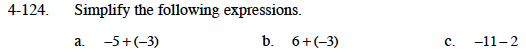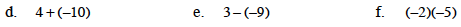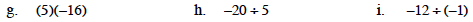### Home > MC1 > Chapter 4 > Lesson 4.3.4 > Problem4-124

4-124.For parts (a), (b), and (c), try drawing a diagram with + and − symbols if you are having difficulty.Parts (d) and (e) are similar to (a) - (c).

Remember that the product of two negative integers is positive.

10If you had 5 groups of 16 negatives, how many negatives would you have?

What would you need to multiply by 5 to get −20?

−4

If you had 12 negatives and you divided them into groups with one negative in each, how many groups would you have?